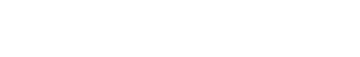#### Revision Notes

##### What do we mean by basic probability?

There topic of probability in mathematics starts off with some very easy concepts but quickly becomes difficult and is one of the hardest topics to fully understand.  Probability is used in many industries as in the real world a lot of predictions or allowing for the unexpected happen. Insurance costs and the rate of interest banks charge on a loan are examples.

##### What do I need to know?
In the first instance you need to be aware of how we write and use probability notation.

1. Event
When we have a trial or experiment there may be several outcomes.
We use capital letters to denote an event – this is something that might happen in our trial. So, for example, A could be the event of getting an even number when rolling a dice. It is more common to see reference to “outcomes” rather than “events” but they broadly mean the same thing.
2. “A-dash”
If A is an event, then A’ (said “A-dash”) is the event “not A”. Notice we’re not necessarily interested in what has happened, just that A hasn’t.
3. Probability of …
P(A) means the probability that event A happens (eg rolling a six on a dice)
P(A’) means the probability that event A does not happen (eg getting any other number when rolling a dice)
The letter n is often used to talk about the number of times A happens (or might happen) if the trial is repeated several times over.
4. Total Probability
All the different events for a trial have a total probability of 1 (certainty). This should make sense in that something will happen from a trial (eg when rolling a (normal) dice it is certain you will get a number between 1 and 6)
5. Experimental Probability
We usually talk about theoretical probability but it is rare you will see that phrase.
This is where the probability of an event can be determined by considering all the possible outcomes (eg on a dice we would say the probability of getting each individual number is ⅙ without performing any trials.
However there are sometimes when we don’t know this (maybe because we know we have a biased dice but don’t know how it is biased) so we can only talk about probabilities once we have done some trials:The more trials that are done, the more the experimental probability will reflect the true probability of the event.
6. Number of outcomes
If we want to estimate the number of times an event will happen out of a total of n trails we calculate:
No. of times A happens = n ×P (A)

You will sometimes see/hear this being called the “expected number of times A happens”.
7. Mutually Exclusive (OR means +)
Mutually exclusive events cannot happen at the same time.
eg Rolling a 2 on a dice and rolling a 4 on a dice
This leads to the result that
P(2) OR P(4) = P(2) + P(4) = ⅙ + ⅙ = 2/6

8. Independent Events (AND means )

This is the first situation where we might combine different events from different trials.

eg A is the event “Rolling a 3” and B is the event “Flipping heads”

These events are independent because one does not affect (the probability of) the other

So to find the probability of both events happening we multiply their individual probabilities together:

P(A) AND P(B) = ⅙ × ½ = ½

Do note that this is NOT the opposite of mutually exclusive – because both often crop up together it is easy to think they must be linked.

#### Example#### Question#### Answer## Want to aim for a Level 9?

See if you’ve got what it takes. Test yourself with our topic questions.### Author: Simon

After 24 years teaching A Level Further Maths, Maths whizz Simon focused his attention on tutoring. He’s helped thousands of young people make sense of Maths and now he’s helping you too – aren’t you lucky! He’s even worked as an Edexcel examiner, so knows exactly what they’re looking for.